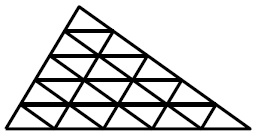# Subdivided triangle

Probability Level 5

A triangle is subdivided into 25 smaller triangles as shown in the figure below. Two of these 25 triangles are selected at random. The probability that the two selected triangles have exactly one vertex in common can be expressed as $\frac{a}{b}$ where $a$ and $b$ are coprime positive integers. What is the value of $a + b$?×